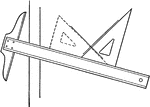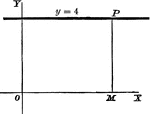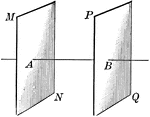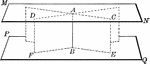Drawing Perpendicular Lines Using T-square and Triangle

Perpendicular lines are drawn by fitting the hypothenuse of the triangle to the T-square at an angle,…Perpendicular Line

A perpendicular line on a coordinate plane, showing what y the y-coordinate will be.Parallel Planes

Illustration of two parallel planes. "Two planes perpendicular to the same straight line are parallel."Parallel Planes

Two parallel planes perpendicular to a line (AB). "A straight line perpendicular to one of two parallel…